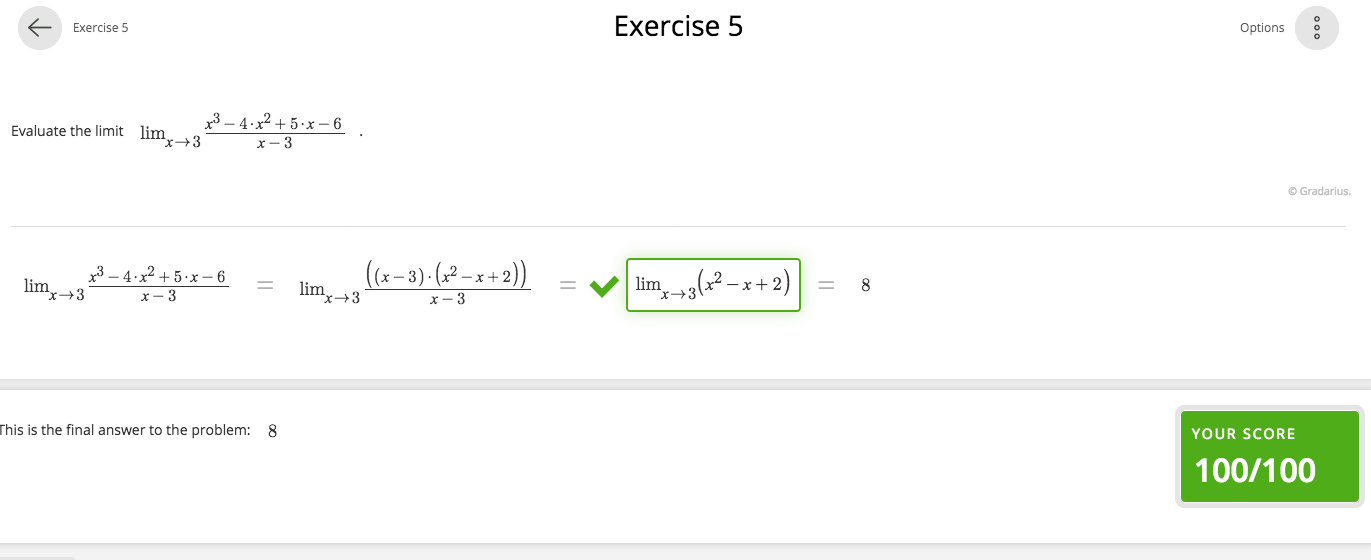Gradarius will give a warning sign as "the higlighted expression is invalid" when you enter multiple equations in a single cell.Gradarius only accepts one equation at a time in a single cell. This is how the input is processed by the system. In general, you can enter two types of mathematical objects into a Gradarius cell, equations and non-equational expressions .  An equation is a mathematical object with an equality sign and an non-equational expressions is any mathematical expression that is not an equation.

If you are solving an equation, note that the following cells in Gradarius must also include equations and they should follow from the previous ones. If you are using a non-equational  expression, then the following expressions must be equivalent to previous ones. Please take a look at the image below to see the proper approach to solving the same problem given as above.